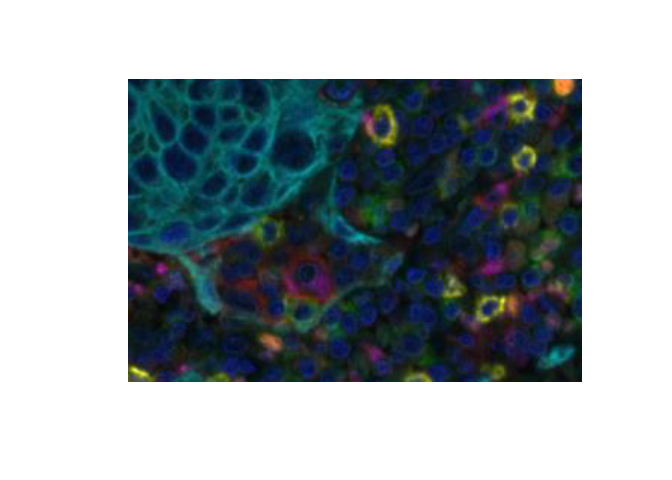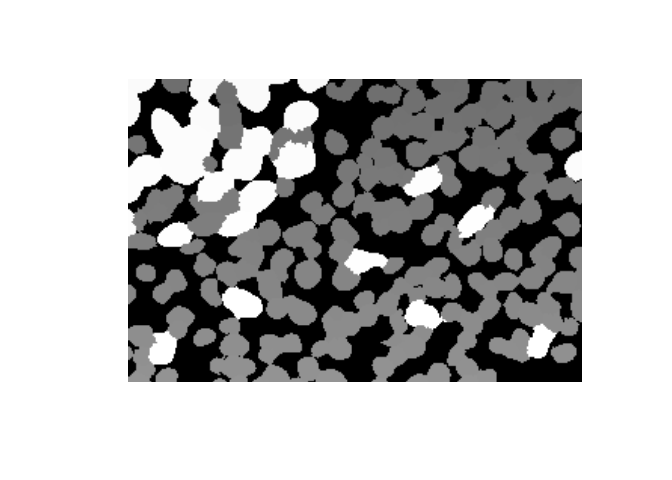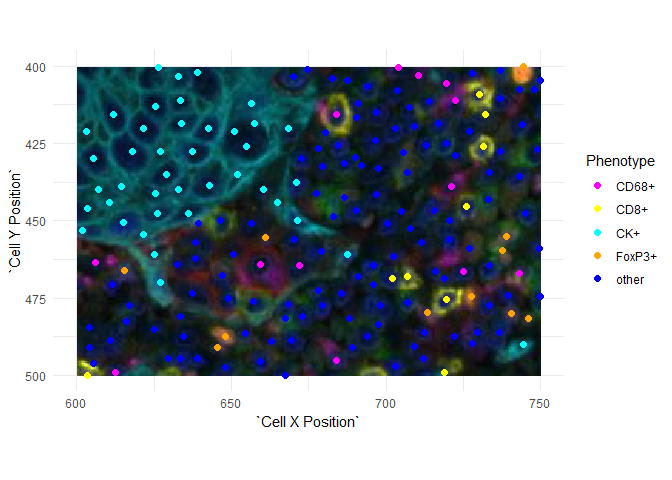inForm writes a variety of image files in JPEG and TIFF format. This tutorial shows how to read the image files in R.

The R packages tiff and jpeg provide functions readTIFF and readJPEG which read image data into R matrices. phenoptr expands on these with read_components and read_maps which read multiple images and extract useful metadata from the Image Description fields.

A variety of functions can display image data including plot.raster, ggplot2::annotation_raster and EBImage::display.

## Coordinate systems

A few items to note:

• Images have the origin (0, 0) at the top left. This is consistent with the Cell X Position and Cell Y Position columns of an inForm cell seg table. The row (first) dimension of an array corresponds to the $$y$$ axis and Cell Y Position column. The column (second) array dimension corresponds to the $$x$$ axis and the Cell X Position column.

• Image dimensions are in pixels. Cell seg tables are written with pixel dimensions and converted to microns when read by phenoptr::read_cell_seg_data.

• Image display methods differ in their orientation; some show $$x$$ and $$y$$ as described above; others transpose the image.

Each of these items easily causes confusion; be careful and verify your work!

## Reading and displaying color images

Color (RGB) images output from inForm, such as the composite image and segmentation images, can be read directly using tiff::readTIFF() or jpeg::readJPEG(). For example,

path <- system.file("extdata", "sample",
"Set4_1-6plex_[16142,55840]_composite_image.jpg",
package = "phenoptr")
dim(img)
 1400 1868    3

Color images are read as 3D arrays. They can be displayed by converting to a raster.

snippet <- as.raster(img[800:1000, 1200:1500,])
plot(snippet)EBImage::display will display an array in a web browser. RGB images are shown as three grayscale planes unless converted to an EBImage::Image first. EBImage::display shows the first coordinate horizontally and the second coordinate vertically so the image must be flipped to display in the expected orientation.

img_transposed <- aperm(img, c(2, 1, 3))
EBImage::display(img_transposed)
EBImage::display(EBImage::Image(img_transposed, colormode='Color'))

## Reading and displaying component data

inForm saves component data as multiple 32-bit floating-point images within a single TIFF file. Images smaller than 2K by 2K pixels are saved in “strip” format and may be read by tiff::readTIFF(). Larger images are saved in “tiled” format which is not supported by readTIFF(). Single fields from Vectra Polaris, Vectra 3 and Mantra are all smaller than 2K by 2K and may be read by these functions; larger fields may not read. Install the Akoya Biosciences fork of the tiff package to support tiled images and remove this limitation.

phenoptr::read_components() is a wrapper around readTIFF() which reads the individual component images from a component_data.tif file. It keeps the full-resolution component images, extracts the component names from the image descriptions, and returns a named list of image matrices.

Component data may be displayed using plot.raster or EBImage::display. See the next section for examples of displaying grayscale images such as these.

## Reading and displaying segmentation maps

inForm saves segmentation maps (nuclear segmentation, etc.) as multiple 16-bit grayscale images within a single TIFF file. Most segmentation images are label images, where each object is represented by a region whose value is the object number. The membrane map is a binary image where the presence of membrane is indicated with a “1” value.

phenoptr::read_maps() is a wrapper around readTIFF() which reads map files. It returns a named list of integer-valued matrices. The list names reflect the content of the individual images, e.g. Nucleus, Cytoplasm, etc.

map_path <- system.file("extdata", "sample",
"Set4_1-6plex_[16142,55840]_binary_seg_maps.tif", package = "phenoptr")
names(maps)
 "Nucleus"   "Cytoplasm" "Membrane"  "Tissue"   

The Nucleus label image contains values for background (0) and each Cell ID.

nucleus <- maps[['Nucleus']]
dim(nucleus)
 1400 1868
range(nucleus)
    0 6183
range(phenoptr::sample_cell_seg_data\$Cell ID)
   11 6183

Label images may be displayed with plot.raster or EBImage::display (which requires swapping axes).

nucleus_snippet <- nucleus[800:1000, 1200:1500]
plot(as.raster(nucleus_snippet, max=max(nucleus_snippet)))EBImage::display(t(nucleus/max(nucleus)))
EBImage::display(t(maps[['Membrane']]))

## Plotting image data

Images have the origin (0, 0) at the top left. This is consistent with the Cell X Position and Cell Y Position columns of an inForm cell seg table but it is reversed (in $$y$$) from the usual plotting conventions. If you plot images and points together, you will have to reverse one or the other.

Here is an example using ggplot2. The $$y$$-axis is reversed using ggplot2::scale_y_reverse() and the image is then un-reversed by negating its $$y$$ limits.

library(ggplot2)
csd <- phenoptr::sample_cell_seg_data
csd <- subset(csd, Cell X Position>=600 & Cell X Position<=750 &
Cell Y Position>=400 & Cell Y Position<=500)
ggplot(data=csd, aes(Cell X Position, Cell Y Position, color=Phenotype)) +
scale_x_continuous(limits=c(600, 750)) +
scale_y_reverse(limits=c(500, 400)) +
annotation_raster(snippet, 600, 750, -400, -500) +
geom_point(size=2) + coord_equal() +
scale_color_manual(values=c("CK+"="cyan", "CD8+"="yellow",
"other"="blue", "CD68+"="magenta",
"FoxP3+"="orange")) +
theme_minimal()